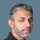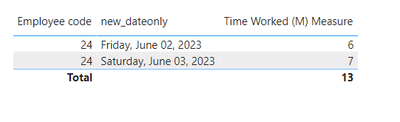cancel
Showing results for
Did you mean:Helper III

## Calculate total hours worked from multiple check in check outs

Hi

I have data in powerbi and i want to calculate the total hours worked by every day and then total for month for each user.

In the report i would liek to select a month and then on top row there will be each day on the left column will be employee code and in cells i want to calculate how many hours emplyee worked for that day. As you see withi na day employee can make multiple chek in and multiple check outs. Can you help me to build this?

My data in powerbi looks like this:1 ACCEPTED SOLUTIONSuper User

@jja

I option is, you create a table that calculates the time in minutes that the employees worked then add a new measure to sum the minutes they worked.

Here is a way to create a table:

``````Table 2 =
FILTER ( table1, Table1[Action] = "OUT" ),
"Time Worked (min)",
VAR __INTIME = Table1[Date and Time]
VAR __EMP = Table1[Employee code]
VAR __DATE = Table1[new_dateonly]
VAR __OUTIME =
MAXX(
FILTER (
ALL(table1), Table1[Employee code] = __EMP && Table1[new_dateonly] = __DATE &&  Table1[Action] = "IN"  && Table1[Date and Time] < __INTIME
),
Table1[Date and Time]
)
VAR __TIMEWORKED = DATEDIFF( __OUTIME , __INTIME ,  MINUTE )
RETURN
__TIMEWORKED
)``````

2nd option is to create a measure, this may be slow depending on our data volume and the model:

``````Time Worked (M) Measure =
VAR __T =
SUMMARIZE (
ALLSELECTED ( Table1 ),
Table1[Employee code],
Table1[new_dateonly],
Table1[Date and Time]
)
RETURN
SUMX (
SUMMARIZE (
FILTER ( Table1, TABLE1[Action] = "IN" ),
Table1[Employee code],
Table1[new_dateonly],
Table1[Date and Time]
),
VAR __INTIME = Table1[Date and Time]
VAR __OUTTIME =
MAXX (
OFFSET (
1,
__T,
ORDERBY ( Table1[Date and Time] ),
PARTITIONBY ( Table1[Employee code], Table1[new_dateonly] )
),
Table1[Date and Time]
)
VAR __WORKED =
DATEDIFF ( __INTIME, __OUTTIME, MINUTE )
RETURN
__WORKED
)``````Did I answer your question? Mark my post as a solution! and hit thumbs up
3 REPLIES 3Helper III

I used as a sollution creating a table and it works as expected untill if user did not chek OUT then the period he worked is not calculated for that day. I would like to return some indication in this case like in best case error or let's say to make a fake OUT records like 23:59 hours(user checked out at midnight) and then calculate time minutes worked. Could you asisst with that as well?Helper III

Thank you

That works perfect!Super User

@jja

I option is, you create a table that calculates the time in minutes that the employees worked then add a new measure to sum the minutes they worked.

Here is a way to create a table:

``````Table 2 =
FILTER ( table1, Table1[Action] = "OUT" ),
"Time Worked (min)",
VAR __INTIME = Table1[Date and Time]
VAR __EMP = Table1[Employee code]
VAR __DATE = Table1[new_dateonly]
VAR __OUTIME =
MAXX(
FILTER (
ALL(table1), Table1[Employee code] = __EMP && Table1[new_dateonly] = __DATE &&  Table1[Action] = "IN"  && Table1[Date and Time] < __INTIME
),
Table1[Date and Time]
)
VAR __TIMEWORKED = DATEDIFF( __OUTIME , __INTIME ,  MINUTE )
RETURN
__TIMEWORKED
)``````

2nd option is to create a measure, this may be slow depending on our data volume and the model:

``````Time Worked (M) Measure =
VAR __T =
SUMMARIZE (
ALLSELECTED ( Table1 ),
Table1[Employee code],
Table1[new_dateonly],
Table1[Date and Time]
)
RETURN
SUMX (
SUMMARIZE (
FILTER ( Table1, TABLE1[Action] = "IN" ),
Table1[Employee code],
Table1[new_dateonly],
Table1[Date and Time]
),
VAR __INTIME = Table1[Date and Time]
VAR __OUTTIME =
MAXX (
OFFSET (
1,
__T,
ORDERBY ( Table1[Date and Time] ),
PARTITIONBY ( Table1[Employee code], Table1[new_dateonly] )
),
Table1[Date and Time]
)
VAR __WORKED =
DATEDIFF ( __INTIME, __OUTTIME, MINUTE )
RETURN
__WORKED
)``````Did I answer your question? Mark my post as a solution! and hit thumbs up